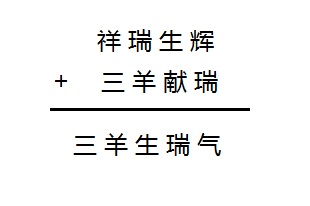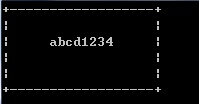# 2015年蓝桥省赛真题B组C++

• 时间:
• 浏览:

### 3. 三羊献瑞(9分)### 4. 格子中输出(11分)

StringInGrid函数会在一个指定大小的格子中打印指定的字符串。

``````下面的程序实现这个逻辑，请填写划线部分缺少的代码。

#include <stdio.h>
#include <string.h>

void StringInGrid(int width, int height, const char* s)
{
int i,k;
char buf;
strcpy(buf, s);
if(strlen(s)>width-2) buf[width-2]=0;

printf("+");
for(i=0;i<width-2;i++) printf("-");
printf("+\n");

for(k=1; k<(height-1)/2;k++){
printf("|");
for(i=0;i<width-2;i++) printf(" ");
printf("|\n");
}

printf("|");

printf("%*s%s%*s",_____________________________________________);  //填空

printf("|\n");

for(k=(height-1)/2+1; k<height-1; k++){
printf("|");
for(i=0;i<width-2;i++) printf(" ");
printf("|\n");
}

printf("+");
for(i=0;i<width-2;i++) printf("-");
printf("+\n");
}

int main()
{
StringInGrid(20,6,"abcd1234");
return 0;
}

+------------------+
|               　　|
|     abcd1234     |
|              　　 |
|             　　  |
+------------------+

``````

（如果出现对齐问题，参看【图1.jpg】）### 5. 九组分数(13分)

``````1,2,3...9 这九个数字组成一个分数，其值恰好为1/3，如何组法？

#include <stdio.h>

void test(int x[])
{
int a = x*1000 + x*100 + x*10 + x;
int b = x*10000 + x*1000 + x*100 + x*10 + x;

if(a*3==b) printf("%d / %d\n", a, b);
}

void f(int x[], int k)
{
int i,t;
if(k>=9){
test(x);
return;
}

for(i=k; i<9; i++){
{t=x[k]; x[k]=x[i]; x[i]=t;}
f(x,k+1);
_____________________________________________ // 填空处
}
}

int main()
{
int x[] = {1,2,3,4,5,6,7,8,9};
f(x,0);
return 0;
}
``````

### 6. 加法变乘法(17分)

1+2+3+…+1011+12+…+2728+29+…+49 = 2015

### 8. 移动距离(15分)

X星球居民小区的楼房全是一样的，并且按矩阵样式排列。其楼房的编号为1,2,3…

1 2 3 4 5 6
12 11 10 9 8 7
13 14 15 …

w为排号宽度，m,n为待计算的楼号。

6 8 2

4

4 7 20

5

### 9. 垒骰子(25分)

atm想计算一下有多少种不同的可能的垒骰子方式。

「输入格式」

n表示骰子数目

「输出格式」

「样例输入」
2 1
1 2

「样例输出」
544

「数据范围」

「输入格式」

「输出格式」

「样例输入」
5
1 -2 -3 4 5
4 2
3 1
1 2
2 5

「样例输出」
8

「数据范围」